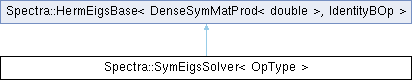Spectra  1.0.1 Header-only C++ Library for Large Scale Eigenvalue Problems
Spectra::SymEigsSolver< OpType > Class Template Reference

#include <Spectra/SymEigsSolver.h>

Inheritance diagram for Spectra::SymEigsSolver< OpType >:## Public Member Functions

SymEigsSolver (OpType &op, Index nev, Index ncv)Public Member Functions inherited from Spectra::SymEigsBase< DenseSymMatProd< double >, IdentityBOp >
void init (const Scalar *init_resid)

void init ()

Index compute (SortRule selection=SortRule::LargestMagn, Index maxit=1000, Scalar tol=1e-10, SortRule sorting=SortRule::LargestAlge)

CompInfo info () const

Index num_iterations () const

Index num_operations () const

Vector eigenvalues () const

virtual Matrix eigenvectors (Index nvec) const

virtual Matrix eigenvectors () const

## Detailed Description

### template<typename OpType = DenseSymMatProd<double>> class Spectra::SymEigsSolver< OpType >

This class implements the eigen solver for real symmetric matrices, i.e., to solve $$Ax=\lambda x$$ where $$A$$ is symmetric.

Spectra is designed to calculate a specified number ( $$k$$) of eigenvalues of a large square matrix ( $$A$$). Usually $$k$$ is much less than the size of the matrix ( $$n$$), so that only a few eigenvalues and eigenvectors are computed.

Rather than providing the whole $$A$$ matrix, the algorithm only requires the matrix-vector multiplication operation of $$A$$. Therefore, users of this solver need to supply a class that computes the result of $$Av$$ for any given vector $$v$$. The name of this class should be given to the template parameter OpType, and instance of this class passed to the constructor of SymEigsSolver.

If the matrix $$A$$ is already stored as a matrix object in Eigen, for example Eigen::MatrixXd, then there is an easy way to construct such a matrix operation class, by using the built-in wrapper class DenseSymMatProd that wraps an existing matrix object in Eigen. This is also the default template parameter for SymEigsSolver. For sparse matrices, the wrapper class SparseSymMatProd can be used similarly.

If the users need to define their own matrix-vector multiplication operation class, it should define a public type Scalar to indicate the element type, and implement all the public member functions as in DenseSymMatProd.

Template Parameters
 OpType The name of the matrix operation class. Users could either use the wrapper classes such as DenseSymMatProd and SparseSymMatProd, or define their own that implements the type definition Scalar and all the public member functions as in DenseSymMatProd.

Below is an example that demonstrates the usage of this class.

#include <Eigen/Core>
#include <Spectra/SymEigsSolver.h>
// <Spectra/MatOp/DenseSymMatProd.h> is implicitly included
#include <iostream>
using namespace Spectra;
int main()
{
// We are going to calculate the eigenvalues of M
Eigen::MatrixXd A = Eigen::MatrixXd::Random(10, 10);
Eigen::MatrixXd M = A + A.transpose();
// Construct matrix operation object using the wrapper class DenseSymMatProd
DenseSymMatProd<double> op(M);
// Construct eigen solver object, requesting the largest three eigenvalues
SymEigsSolver<DenseSymMatProd<double>> eigs(op, 3, 6);
// Initialize and compute
eigs.init();
int nconv = eigs.compute(SortRule::LargestAlge);
// Retrieve results
Eigen::VectorXd evalues;
if (eigs.info() == CompInfo::Successful)
evalues = eigs.eigenvalues();
std::cout << "Eigenvalues found:\n" << evalues << std::endl;
return 0;
}
@ Successful
Computation was successful.

And here is an example for user-supplied matrix operation class.

#include <Eigen/Core>
#include <Spectra/SymEigsSolver.h>
#include <iostream>
using namespace Spectra;
// M = diag(1, 2, ..., 10)
class MyDiagonalTen
{
public:
using Scalar = double; // A typedef named "Scalar" is required
int rows() const { return 10; }
int cols() const { return 10; }
// y_out = M * x_in
void perform_op(double *x_in, double *y_out) const
{
for (int i = 0; i < rows(); i++)
{
y_out[i] = x_in[i] * (i + 1);
}
}
};
int main()
{
MyDiagonalTen op;
SymEigsSolver<MyDiagonalTen> eigs(op, 3, 6);
eigs.init();
eigs.compute(SortRule::LargestAlge);
if (eigs.info() == CompInfo::Successful)
{
Eigen::VectorXd evalues = eigs.eigenvalues();
// Will get (10, 9, 8)
std::cout << "Eigenvalues found:\n" << evalues << std::endl;
}
return 0;
}

Definition at line 134 of file SymEigsSolver.h.

## ◆ SymEigsSolver()

template<typename OpType = DenseSymMatProd<double>>
 Spectra::SymEigsSolver< OpType >::SymEigsSolver ( OpType & op, Index nev, Index ncv )
inline

Constructor to create a solver object.

Parameters
 op The matrix operation object that implements the matrix-vector multiplication operation of $$A$$: calculating $$Av$$ for any vector $$v$$. Users could either create the object from the wrapper class such as DenseSymMatProd, or define their own that implements all the public members as in DenseSymMatProd. nev Number of eigenvalues requested. This should satisfy $$1\le nev \le n-1$$, where $$n$$ is the size of matrix. ncv Parameter that controls the convergence speed of the algorithm. Typically a larger ncv means faster convergence, but it may also result in greater memory use and more matrix operations in each iteration. This parameter must satisfy $$nev < ncv \le n$$, and is advised to take $$ncv \ge 2\cdot nev$$.

Definition at line 157 of file SymEigsSolver.h.

The documentation for this class was generated from the following file: